### Upon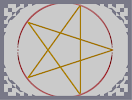Hover over the thumbnail for a full-size version.

Author bobshike author:bobshike bitesized n-art rated 2006-03-16 2006-03-16 4 by 42 people. \$Upon#bobshike#none#100M0M0I0000000F0J0J0010103I0000000000000F401000550000000000000002200E350000000000000000024B0A0000000000000000000>0E000000000000000000000B00000000000000000000000A000000000000000000000>000000000000000000000000000000000000000000000000000000000000000000000000000000000000000000000000000000000000000000000000000000000000000000000000000000000000000000000000000000000000000000000000000000000000000000000000000000000000000000000000000000000000000000000000000000000000000000000000000000000000000000000000000000000000000000000000000000000@000000000000000000000?00000000000000000000000D000000000000000000000C0@0000000000000000000?0D240000000000000000035C004400000000000000033000102H0000000000000G5010100L0L0H0000000G0K0K001|0^168,134.6351053416!0^168,465.3648946384!0^169,134.9600250378!0^169,465.0399749422!0^170,135.284944734!0^170,464.715055246!0^171,135.6098644302!0^171,464.3901355498!0^172,135.9347841264!0^172,464.0652158536!0^173,136.2597038226!0^173,463.7402961574!0^174,136.5846235188!0^174,463.4153764612!0^175,136.909543215!0^175,463.090456765!0^176,137.2344629112!0^176,462.7655370688!0^177,137.5593826074!0^177,462.4406173726!0^178,137.8843023036!0^178,462.1156976764!0^179,138.2092219998!0^179,461.7907779802!0^180,138.534141696!0^180,461.465858284!0^181,138.8590613922!0^181,461.1409385878!0^182,139.1839810884!0^182,460.8160188916!0^183,139.5089007846!0^183,460.4910991954!0^184,139.8338204808!0^184,460.1661794992!0^185,140.158740177!0^185,459.841259803!0^186,140.4836598732!0^186,459.5163401068!0^187,140.8085795694!0^187,459.1914204106!0^188,141.1334992656!0^188,458.8665007144!0^189,141.4584189618!0^189,458.5415810182!0^190,141.783338658!0^190,458.216661322!0^191,142.1082583542!0^191,457.8917416258!0^192,142.4331780504!0^192,457.5668219296!0^193,142.7580977466!0^193,457.2419022334!0^194,143.0830174428!0^194,456.9169825372!0^195,143.407937139!0^195,456.592062841!0^196,143.7328568352!0^196,456.2671431448!0^197,144.0577765314!0^197,455.9422234486!0^198,144.3826962276!0^198,455.6173037524!0^199,144.7076159238!0^199,455.2923840562!0^200,145.03253562!0^200,454.96746436!0^201,145.3574553162!0^201,454.6425446638!0^202,145.6823750124!0^202,454.3176249676!0^203,146.0072947086!0^203,453.9927052714!0^204,146.3322144048!0^204,453.6677855752!0^205,146.657134101!0^205,453.342865879!0^206,146.9820537972!0^206,453.0179461828!0^207,147.3069734934!0^207,452.6930264866!0^208,147.6318931896!0^208,452.3681067904!0^209,147.9568128858!0^209,452.0431870942!0^210,148.281732582!0^210,451.718267398!0^211,148.6066522782!0^211,451.3933477018!0^212,148.9315719744!0^212,451.0684280056!0^213,149.2564916706!0^213,450.7435083094!0^214,149.5814113668!0^214,450.4185886132!0^215,149.906331063!0^215,450.093668917!0^216,150.2312507592!0^216,449.7687492208!0^217,150.5561704554!0^217,449.4438295246!0^218,150.8810901516!0^218,449.1189098284!0^219,151.2060098478!0^219,448.7939901322!0^220,151.530929544!0^220,448.469070436!0^221,151.8558492402!0^221,448.1441507398!0^222,152.1807689364!0^222,447.8192310436!0^223,152.5056886326!0^223,447.4943113474!0^224,152.8306083288!0^224,447.1693916512!0^225,153.155528025!0^225,446.844471955!0^226,153.4804477212!0^226,446.5195522588!0^227,153.8053674174!0^227,446.1946325626!0^228,154.1302871136!0^228,445.8697128664!0^229,154.4552068098!0^229,445.5447931702!0^230,154.780126506!0^230,445.219873474!0^231,155.1050462022!0^231,444.8949537778!0^232,155.4299658984!0^232,444.5700340816!0^233,155.7548855946!0^233,444.2451143854!0^234,156.0798052908!0^234,443.9201946892!0^235,156.404724987!0^235,443.595274993!0^236,156.7296446832!0^236,443.2703552968!0^237,157.0545643794!0^237,442.9454356006!0^238,157.3794840756!0^238,442.6205159044!0^239,157.7044037718!0^239,442.2955962082!0^240,158.029323468!0^240,441.970676512!0^241,158.3542431642!0^241,441.6457568158!0^242,158.6791628604!0^242,441.3208371196!0^243,159.0040825566!0^243,440.9959174234!0^244,159.3290022528!0^244,440.6709977272!0^245,159.653921949!0^245,440.346078031!0^246,159.9788416452!0^246,440.0211583348!0^247,160.3037613414!0^247,439.6962386386!0^248,160.6286810376!0^248,439.3713189424!0^249,160.9536007338!0^249,439.0463992462!0^250,161.27852043!0^250,438.72147955!0^251,161.6034401262!0^251,438.3965598538!0^252,161.9283598224!0^252,438.0716401576!0^253,162.2532795186!0^253,437.7467204614!0^254,162.5781992148!0^254,437.4218007652!0^255,162.903118911!0^255,437.096881069!0^256,163.2280386072!0^256,436.7719613728!0^257,163.5529583034!0^257,436.4470416766!0^258,163.8778779996!0^258,436.1221219804!0^259,164.2027976958!0^259,435.7972022842!0^260,164.527717392!0^260,435.472282588!0^261,164.8526370882!0^261,435.1473628918!0^262,165.1775567844!0^262,434.8224431956!0^263,165.5024764806!0^263,434.4975234994!0^264,165.8273961768!0^264,434.1726038032!0^265,166.152315873!0^265,433.847684107!0^266,166.4772355692!0^266,433.5227644108!0^267,166.8021552654!0^267,433.1978447146!0^268,167.1270749616!0^268,432.8729250184!0^269,167.4519946578!0^269,432.5480053222!0^270,167.776914354!0^270,432.223085626!0^271,168.1018340502!0^271,431.8981659298!0^272,168.4267537464!0^272,431.5732462336!0^273,168.7516734426!0^273,431.2483265374!0^274,169.0765931388!0^274,430.9234068412!0^275,169.401512835!0^275,430.598487145!0^276,169.7264325312!0^276,430.2735674488!0^277,170.0513522274!0^277,429.9486477526!0^278,170.3762719236!0^278,429.6237280564!0^279,170.7011916198!0^279,429.2988083602!0^280,171.026111316!0^280,428.973888664!0^281,171.3510310122!0^281,428.6489689678!0^282,171.6759507084!0^282,428.3240492716!0^283,172.0008704046!0^283,427.9991295754!0^284,172.3257901008!0^284,427.6742098792!0^285,172.650709797!0^285,427.349290183!0^286,172.9756294932!0^286,427.0243704868!0^287,173.3005491894!0^287,426.6994507906!0^288,173.6254688856!0^288,426.3745310944!0^289,173.9503885818!0^289,426.0496113982!0^290,174.275308278!0^290,425.724691702!0^291,174.6002279742!0^291,425.3997720058!0^292,174.9251476704!0^292,425.0748523096!0^293,175.2500673666!0^293,424.7499326134!0^294,175.5749870628!0^294,424.4250129172!0^295,175.899906759!0^295,424.100093221!0^296,176.2248264552!0^296,423.7751735248!0^297,176.5497461514!0^297,423.4502538286!0^298,176.8746658476!0^298,423.1253341324!0^299,177.1995855438!0^299,422.8004144362!0^300,177.52450524!0^300,422.47549474!0^301,177.8494249362!0^301,422.1505750438!0^302,178.1743446324!0^302,421.8256553476!0^303,178.4992643286!0^303,421.5007356514!0^304,178.8241840248!0^304,421.1758159552!0^305,179.149103721!0^305,420.850896259!0^306,179.4740234172!0^306,420.5259765628!0^307,179.7989431134!0^307,420.2010568666!0^308,180.1238628096!0^308,419.8761371704!0^309,180.4487825058!0^309,419.5512174742!0^310,180.773702202!0^310,419.226297778!0^311,181.0986218982!0^311,418.9013780818!0^312,181.4235415944!0^312,418.5764583856!0^313,181.7484612906!0^313,418.2515386894!0^314,182.0733809868!0^314,417.9266189932!0^315,182.398300683!0^315,417.601699297!0^316,182.7232203792!0^316,417.2767796008!0^317,183.0481400754!0^317,416.9518599046!0^318,183.3730597716!0^318,416.6269402084!0^319,183.6979794678!0^319,416.3020205122!0^320,184.022899164!0^320,415.977100816!0^321,184.3478188602!0^321,415.6521811198!0^322,184.6727385564!0^322,415.3272614236!0^323,184.9976582526!0^323,415.0023417274!0^324,185.3225779488!0^324,414.6774220312!0^325,185.647497645!0^325,414.352502335!0^326,185.9724173412!0^326,414.0275826388!0^327,186.2973370374!0^327,413.7026629426!0^328,186.6222567336!0^328,413.3777432464!0^329,186.9471764298!0^329,413.0528235502!0^330,187.272096126!0^330,412.727903854!0^331,187.5970158222!0^331,412.4029841578!0^332,187.9219355184!0^332,412.0780644616!0^333,188.2468552146!0^333,411.7531447654!0^334,188.5717749108!0^334,411.4282250692!0^335,188.896694607!0^335,411.103305373!0^336,189.2216143032!0^336,410.7783856768!0^337,189.5465339994!0^337,410.4534659806!0^338,189.8714536956!0^338,410.1285462844!0^339,190.1963733918!0^339,409.8036265882!0^340,190.521293088!0^340,409.478706892!0^341,190.8462127842!0^341,409.1537871958!0^342,191.1711324804!0^342,408.8288674996!0^343,191.4960521766!0^343,408.5039478034!0^344,191.8209718728!0^344,408.1790281072!0^345,192.145891569!0^345,407.854108411!0^346,192.4708112652!0^346,407.5291887148!0^347,192.7957309614!0^347,407.2042690186!0^348,193.1206506576!0^348,406.8793493224!0^349,193.4455703538!0^349,406.5544296262!0^350,193.77049005!0^350,406.22950993!0^351,194.0954097462!0^351,405.9045902338!0^352,194.4203294424!0^352,405.5796705376!0^353,194.7452491386!0^353,405.2547508414!0^354,195.0701688348!0^354,404.9298311452!0^355,195.395088531!0^355,404.604911449!0^356,195.7200082272!0^356,404.2799917528!0^357,196.0449279234!0^357,403.9550720566!0^358,196.3698476196!0^358,403.6301523604!0^359,196.6947673158!0^359,403.3052326642!0^360,197.019687012!0^360,402.980312968!0^361,197.3446067082!0^361,402.6553932718!0^362,197.6695264044!0^362,402.3304735756!0^363,197.9944461006!0^363,402.0055538794!0^364,198.3193657968!0^364,401.6806341832!0^365,198.644285493!0^365,401.355714487!0^366,198.9692051892!0^366,401.0307947908!0^367,199.2941248854!0^367,400.7058750946!0^368,199.6190445816!0^368,400.3809553984!0^369,199.9439642778!0^369,400.0560357022!0^370,200.268883974!0^370,399.731116006!0^371,200.5938036702!0^371,399.4061963098!0^372,200.9187233664!0^372,399.0812766136!0^373,201.2436430626!0^373,398.7563569174!0^374,201.5685627588!0^374,398.4314372212!0^375,201.893482455!0^375,398.106517525!0^376,202.2184021512!0^376,397.7815978288!0^377,202.5433218474!0^377,397.4566781326!0^378,202.8682415436!0^378,397.1317584364!0^379,203.1931612398!0^379,396.8068387402!0^380,203.518080936!0^380,396.481919044!0^381,203.8430006322!0^381,396.1569993478!0^382,204.1679203284!0^382,395.8320796516!0^383,204.4928400246!0^383,395.5071599554!0^384,204.8177597208!0^384,395.1822402592!0^385,205.142679417!0^385,394.857320563!0^386,205.4675991132!0^386,394.5324008668!0^387,205.7925188094!0^387,394.2074811706!0^388,206.1174385056!0^388,393.8825614744!0^389,206.4423582018!0^389,393.5576417782!0^390,206.767277898!0^390,393.232722082!0^391,207.0921975942!0^391,392.9078023858!0^392,207.4171172904!0^392,392.5828826896!0^393,207.7420369866!0^393,392.2579629934!0^394,208.0669566828!0^394,391.9330432972!0^395,208.391876379!0^395,391.608123601!0^396,208.7167960752!0^396,391.2832039048!0^397,209.0417157714!0^397,390.9582842086!0^398,209.3666354676!0^398,390.6333645124!0^399,209.6915551638!0^399,390.3084448162!0^400,210.01647486!0^400,389.98352512!0^401,210.3413945562!0^401,389.6586054238!0^402,210.6663142524!0^402,389.3336857276!0^403,210.9912339486!0^403,389.0087660314!0^404,211.3161536448!0^404,388.6838463352!0^405,211.641073341!0^405,388.358926639!0^406,211.9659930372!0^406,388.0340069428!0^407,212.2909127334!0^407,387.7090872466!0^408,212.6158324296!0^408,387.3841675504!0^409,212.9407521258!0^409,387.0592478542!0^410,213.265671822!0^410,386.734328158!0^411,213.5905915182!0^411,386.4094084618!0^412,213.9155112144!0^412,386.0844887656!0^413,214.2404309106!0^413,385.7595690694!0^414,214.5653506068!0^414,385.4346493732!0^415,214.890270303!0^415,385.109729677!0^416,215.2151899992!0^416,384.7848099808!0^417,215.5401096954!0^417,384.4598902846!0^418,215.8650293916!0^418,384.1349705884!0^419,216.1899490878!0^419,383.8100508922!0^420,216.514868784!0^420,383.485131196!0^421,216.8397884802!0^421,383.1602114998!0^422,217.1647081764!0^422,382.8352918036!0^423,217.4896278726!0^423,382.5103721074!0^424,217.8145475688!0^424,382.1854524112!0^425,218.139467265!0^425,381.860532715!0^426,218.4643869612!0^426,381.5356130188!0^427,218.7893066574!0^427,381.2106933226!0^428,219.1142263536!0^428,380.8857736264!0^429,219.4391460498!0^429,380.5608539302!0^430,219.764065746!0^430,380.235934234!0^431,220.0889854422!0^431,379.9110145378!0^432,220.4139051384!0^432,379.5860948416!0^433,220.7388248346!0^433,379.2611751454!0^434,221.0637445308!0^434,378.9362554492!0^435,221.388664227!0^435,378.611335753!0^436,221.7135839232!0^436,378.2864160568!0^437,222.0385036194!0^437,377.9614963606!0^438,222.3634233156!0^438,377.6365766644!0^439,222.6883430118!0^439,377.3116569682!0^440,223.013262708!0^440,376.986737272!0^441,223.3381824042!0^441,376.6618175758!0^442,223.6631021004!0^442,376.3368978796!0^443,223.9880217966!0^443,376.0119781834!0^444,224.3129414928!0^444,375.6870584872!0^445,224.637861189!0^445,375.362138791!0^446,224.9627808852!0^446,375.0372190948!0^447,225.2877005814!0^447,374.7122993986!0^448,225.6126202776!0^448,374.3873797024!0^449,225.9375399738!0^449,374.0624600062!0^450,226.26245967!0^450,373.73754031!0^451,226.5873793662!0^451,373.4126206138!0^452,226.9122990624!0^452,373.0877009176!0^453,227.2372187586!0^453,372.7627812214!0^454,227.5621384548!0^454,372.4378615252!0^455,227.887058151!0^455,372.112941829!0^456,228.2119778472!0^456,371.7880221328!0^457,228.5368975434!0^457,371.4631024366!0^458,228.8618172396!0^458,371.1381827404!0^459,229.1867369358!0^459,370.8132630442!0^460,229.511656632!0^460,370.488343348!0^461,229.8365763282!0^461,370.1634236518!0^462,230.1614960244!0^462,369.8385039556!0^463,230.4864157206!0^463,369.5135842594!0^464,230.8113354168!0^464,369.1886645632!0^465,231.136255113!0^465,368.863744867!0^466,231.4611748092!0^466,368.5388251708!0^467,231.7860945054!0^467,368.2139054746!0^468,232.1110142016!0^468,367.8889857784!0^469,232.4359338978!0^469,367.5640660822!0^470,232.760853594!0^470,367.239146386!0^471,233.0857732902!0^471,366.9142266898!0^472,233.4106929864!0^472,366.5893069936!0^473,233.7356126826!0^473,366.2643872974!0^474,234.0605323788!0^474,365.9394676012!0^475,234.385452075!0^475,365.614547905!0^476,234.7103717712!0^476,365.2896282088!0^477,235.0352914674!0^477,364.9647085126!0^478,235.3602111636!0^478,364.6397888164!0^479,235.6851308598!0^479,364.3148691202!0^480,236.010050556!0^480,363.989949424!0^481,236.3349702522!0^481,363.6650297278!0^482,236.6598899484!0^482,363.3401100316!0^483,236.9848096446!0^483,363.0151903354!0^484,237.3097293408!0^484,362.6902706392!0^485,237.634649037!0^485,362.365350943!0^486,237.9595687332!0^486,362.0404312468!0^487,238.2844884294!0^487,361.7155115506!0^488,238.6094081256!0^488,361.3905918544!0^489,238.9343278218!0^489,361.0656721582!0^490,239.259247518!0^490,360.740752462!0^491,239.5841672142!0^491,360.4158327658!0^492,239.9090869104!0^492,360.0909130696!0^493,240.2340066066!0^493,359.7659933734!0^494,240.5589263028!0^494,359.4410736772!0^495,240.883845999!0^495,359.116153981!0^496,241.2087656952!0^496,358.7912342848!0^497,241.5336853914!0^497,358.4663145886!0^498,241.8586050876!0^498,358.1413948924!0^499,242.1835247838!0^499,357.8164751962!0^500,242.50844448!0^500,357.4915555!0^501,242.8333641762!0^501,357.1666358038!0^502,243.1582838724!0^502,356.8417161076!0^503,243.4832035686!0^503,356.5167964114!0^504,243.8081232648!0^504,356.1918767152!0^505,244.133042961!0^505,355.866957019!0^506,244.4579626572!0^506,355.5420373228!0^507,244.7828823534!0^507,355.2171176266!0^508,245.1078020496!0^508,354.8921979304!0^509,245.4327217458!0^509,354.5672782342!0^510,245.757641442!0^510,354.242358538!0^511,246.0825611382!0^511,353.9174388418!0^512,246.4074808344!0^512,353.5925191456!0^513,246.7324005306!0^513,353.2675994494!0^514,247.0573202268!0^514,352.9426797532!0^515,247.382239923!0^515,352.617760057!0^516,247.7071596192!0^516,352.2928403608!0^517,248.0320793154!0^517,351.9679206646!0^518,248.3569990116!0^518,351.6430009684!0^519,248.6819187078!0^519,351.3180812722!0^520,249.006838404!0^520,350.993161576!0^521,249.3317581002!0^521,350.6682418798!0^522,249.6566777964!0^522,350.3433221836!0^523,249.9815974926!0^523,350.0184024874!0^524,250.3065171888!0^524,349.6934827912!0^525,250.631436885!0^525,349.368563095!0^526,250.9563565812!0^526,349.0436433988!0^527,251.2812762774!0^527,348.7187237026!0^528,251.6061959736!0^528,348.3938040064!0^529,251.9311156698!0^529,348.0688843102!0^530,252.256035366!0^530,347.743964614!0^531,252.5809550622!0^531,347.4190449178!0^532,252.9058747584!0^532,347.0941252216!0^533,253.2307944546!0^533,346.7692055254!0^534,253.5557141508!0^534,346.4442858292!0^535,253.880633847!0^535,346.119366133!0^536,254.2055535432!0^536,345.7944464368!0^537,254.5304732394!0^537,345.4695267406!0^538,254.8553929356!0^538,345.1446070444!0^539,255.1803126318!0^539,344.8196873482!0^540,255.505232328!0^540,344.494767652!0^541,255.8301520242!0^541,344.1698479558!0^542,256.1550717204!0^542,343.8449282596!0^543,256.4799914166!0^543,343.5200085634!0^544,256.8049111128!0^544,343.1950888672!0^545,257.129830809!0^545,342.870169171!0^546,257.4547505052!0^546,342.5452494748!0^547,257.7796702014!0^547,342.2203297786!0^548,258.1045898976!0^548,341.8954100824!0^549,258.4295095938!0^549,341.5704903862!0^550,258.75442929!0^550,341.24557069!0^551,259.0793489862!0^551,340.9206509938!0^552,259.4042686824!0^552,340.5957312976!0^553,259.7291883786!0^553,340.2708116014!0^554,260.0541080748!0^554,339.9458919052!0^555,260.379027771!0^555,339.620972209!0^556,260.7039474672!0^556,339.2960525128!0^557,261.0288671634!0^557,338.9711328166!0^558,261.3537868596!0^558,338.6462131204!0^559,261.6787065558!0^559,338.3212934242!0^560,262.003626252!0^560,337.996373728!0^561,262.3285459482!0^561,337.6714540318!0^562,262.6534656444!0^562,337.3465343356!0^563,262.9783853406!0^563,337.0216146394!0^564,263.3033050368!0^564,336.6966949432!0^565,263.628224733!0^565,336.371775247!0^566,263.9531444292!0^566,336.0468555508!0^567,264.2780641254!0^567,335.7219358546!0^568,264.6029838216!0^568,335.3970161584!0^569,264.9279035178!0^569,335.0720964622!0^570,265.252823214!0^570,334.747176766!0^571,265.5777429102!0^571,334.4222570698!0^572,265.9026626064!0^572,334.0973373736!0^573,266.2275823026!0^573,333.7724176774!0^574,266.5525019988!0^574,333.4474979812!0^575,266.877421695!0^575,333.122578285!0^576,267.2023413912!0^576,332.7976585888!0^577,267.5272610874!0^577,332.4727388926!0^578,267.8521807836!0^578,332.1478191964!0^579,268.1771004798!0^579,331.8228995002!0^580,268.502020176!0^580,331.497979804!0^581,268.8269398722!0^581,331.1730601078!0^582,269.1518595684!0^582,330.8481404116!0^583,269.4767792646!0^583,330.5232207154!0^584,269.8016989608!0^584,330.1983010192!0^585,270.126618657!0^585,329.873381323!0^586,270.4515383532!0^586,329.5484616268!0^587,270.7764580494!0^587,329.2235419306!0^588,271.1013777456!0^588,328.8986222344!0^589,271.4262974418!0^589,328.5737025382!0^590,271.751217138!0^590,328.248782842!0^591,272.0761368342!0^591,327.9238631458!0^592,272.4010565304!0^592,327.5989434496!0^593,272.7259762266!0^593,327.2740237534!0^594,273.0508959228!0^594,326.9491040572!0^595,273.375815619!0^595,326.624184361!0^596,273.7007353152!0^596,326.2992646648!0^597,274.0256550114!0^597,325.9743449686!0^598,274.3505747076!0^598,325.6494252724!0^599,274.6754944038!0^599,325.3245055762!0^600,275.0004141!0^600,324.99958588!0^601,275.3253337962!0^601,324.6746661838!0^602,275.6502534924!0^602,324.3497464876!0^603,275.9751731886!0^603,324.0248267914!0^604,276.3000928848!0^604,323.6999070952!0^605,276.625012581!0^605,323.374987399!0^606,276.9499322772!0^606,323.0500677028!0^607,277.2748519734!0^607,322.7251480066!0^608,277.5997716696!0^608,322.4002283104!0^609,277.9246913658!0^609,322.0753086142!0^610,278.249611062!0^610,321.750388918!0^611,278.5745307582!0^611,321.4254692218!0^612,278.8994504544!0^612,321.1005495256!0^613,279.2243701506!0^613,320.7756298294!0^614,279.5492898468!0^614,320.4507101332!0^615,279.874209543!0^615,320.125790437!0^616,280.1991292392!0^616,319.8008707408!0^617,280.5240489354!0^617,319.4759510446!0^618,280.8489686316!0^618,319.1510313484!0^619,281.1738883278!0^619,318.8261116522!0^620,281.498808024!0^620,318.501191956!0^621,281.8237277202!0^621,318.1762722598!0^622,282.1486474164!0^622,317.8513525636!0^623,282.4735671126!0^623,317.5264328674!0^624,282.7984868088!0^624,317.2015131712!0^625,283.123406505!0^625,316.876593475!0^626,283.4483262012!0^626,316.5516737788!0^627,283.7732458974!0^627,316.2267540826!0^628,284.0981655936!0^628,315.9018343864!0^629,284.4230852898!0^629,315.5769146902!0^630,284.748004986!0^630,315.251994994!0^631,285.0729246822!0^631,314.9270752978!0^632,285.3978443784!0^632,314.6021556016!0^633,285.7227640746!0^633,314.2772359054!0^634,286.0476837708!0^634,313.9523162092!0^635,286.372603467!0^635,313.627396513!0^636,286.6975231632!0^636,313.3024768168!0^637,287.0224428594!0^637,312.9775571206!0^638,287.3473625556!0^638,312.6526374244!0^639,287.6722822518!0^639,312.3277177282!0^640,287.997201948!0^640,312.002798032!0^641,288.3221216442!0^641,311.6778783358!0^642,288.6470413404!0^642,311.3529586396!0^643,288.9719610366!0^643,311.0280389434!0^644,289.2968807328!0^644,310.7031192472!0^645,289.621800429!0^645,310.378199551!0^646,289.9467201252!0^646,310.0532798548!0^647,290.2716398214!0^647,309.7283601586!0^648,290.5965595176!0^648,309.4034404624!0^649,290.9214792138!0^649,309.0785207662!0^650,291.24639891!0^650,308.75360107!0^651,291.5713186062!0^651,308.4286813738!0^652,291.8962383024!0^652,308.1037616776!0^653,292.2211579986!0^653,307.7788419814!0^654,292.5460776948!0^654,307.4539222852!0^655,292.870997391!0^655,307.129002589!0^656,293.1959170872!0^656,306.8040828928!0^657,293.5208367834!0^657,306.4791631966!0^658,293.8457564796!0^658,306.1542435004!0^659,294.1706761758!0^659,305.8293238042!0^660,294.495595872!0^660,305.504404108!0^661,294.8205155682!0^661,305.1794844118!0^662,295.1454352644!0^662,304.8545647156!0^663,295.4703549606!0^663,304.5296450194!0^664,295.7952746568!0^664,304.2047253232!0^665,296.120194353!0^665,303.879805627!0^666,296.4451140492!0^666,303.5548859308!0^667,296.7700337454!0^667,303.2299662346!0^668,297.0949534416!0^668,302.9050465384!0^669,297.4198731378!0^669,302.5801268422!0^670,297.744792834!0^670,302.255207146!0^671,298.0697125302!0^671,301.9302874498!0^672,298.3946322264!0^672,301.6053677536!0^673,298.7195519226!0^673,301.2804480574!0^674,299.0444716188!0^674,300.9555283612!0^675,299.369391315!0^675,300.630608665!0^676,299.6943110112!0^676,300.3056889688!0^168,133.877861456!0^168,466.122138544!0^169,135.263187648!0^169,464.736812352!0^170,136.64851384!0^170,463.35148616!0^171,138.033840032!0^171,461.966159968!0^172,139.419166224!0^172,460.580833776!0^173,140.804492416!0^173,459.195507584!0^174,142.189818608!0^174,457.810181392!0^175,143.5751448!0^175,456.4248552!0^176,144.960470992!0^176,455.039529008!0^177,146.345797184!0^177,453.654202816!0^178,147.731123376!0^178,452.268876624!0^179,149.116449568!0^179,450.883550432!0^180,150.50177576!0^180,449.49822424!0^181,151.887101952!0^181,448.112898048!0^182,153.272428144!0^182,446.727571856!0^183,154.657754336!0^183,445.342245664!0^184,156.043080528!0^184,443.956919472!0^185,157.42840672!0^185,442.57159328!0^186,158.813732912!0^186,441.186267088!0^187,160.199059104!0^187,439.800940896!0^188,161.584385296!0^188,438.415614704!0^189,162.969711488!0^189,437.030288512!0^190,164.35503768!0^190,435.64496232!0^191,165.740363872!0^191,434.259636128!0^192,167.125690064!0^192,432.874309936!0^193,168.511016256!0^193,431.488983744!0^194,169.896342448!0^194,430.103657552!0^195,171.28166864!0^195,428.71833136!0^196,172.666994832!0^196,427.333005168!0^197,174.052321024!0^197,425.947678976!0^198,175.437647216!0^198,424.562352784!0^199,176.822973408!0^199,423.177026592!0^200,178.2082996!0^200,421.7917004!0^201,179.593625792!0^201,420.406374208!0^202,180.978951984!0^202,419.021048016!0^203,182.364278176!0^203,417.635721824!0^204,183.749604368!0^204,416.250395632!0^205,185.13493056!0^205,414.86506944!0^206,186.520256752!0^206,413.479743248!0^207,187.905582944!0^207,412.094417056!0^208,189.290909136!0^208,410.709090864!0^209,190.676235328!0^209,409.323764672!0^210,192.06156152!0^210,407.93843848!0^211,193.446887712!0^211,406.553112288!0^212,194.832213904!0^212,405.167786096!0^213,196.217540096!0^213,403.782459904!0^214,197.602866288!0^214,402.397133712!0^215,198.98819248!0^215,401.01180752!0^216,200.373518672!0^216,399.626481328!0^217,201.758844864!0^217,398.241155136!0^218,203.144171056!0^218,396.855828944!0^219,204.529497248!0^219,395.470502752!0^220,205.91482344!0^220,394.08517656!0^221,207.300149632!0^221,392.699850368!0^222,208.685475824!0^222,391.314524176!0^223,210.070802016!0^223,389.929197984!0^224,211.456128208!0^224,388.543871792!0^225,212.8414544!0^225,387.1585456!0^226,214.226780592!0^226,385.773219408!0^227,215.612106784!0^227,384.387893216!0^228,216.997432976!0^228,383.002567024!0^229,218.382759168!0^229,381.617240832!0^230,219.76808536!0^230,380.23191464!0^231,221.153411552!0^231,378.846588448!0^232,222.538737744!0^232,377.461262256!0^233,223.924063936!0^233,376.075936064!0^234,225.309390128!0^234,374.690609872!0^235,226.69471632!0^235,373.30528368!0^236,228.080042512!0^236,371.919957488!0^237,229.465368704!0^237,370.534631296!0^238,230.850694896!0^238,369.149305104!0^239,232.236021088!0^239,367.763978912!0^240,233.62134728!0^240,366.37865272!0^241,235.006673472!0^241,364.993326528!0^242,236.391999664!0^242,363.608000336!0^243,237.777325856!0^243,362.222674144!0^244,239.162652048!0^244,360.837347952!0^245,240.54797824!0^245,359.45202176!0^246,241.933304432!0^246,358.066695568!0^247,243.318630624!0^247,356.681369376!0^248,244.703956816!0^248,355.296043184!0^249,246.089283008!0^249,353.910716992!0^250,247.4746092!0^250,352.5253908!0^251,248.859935392!0^251,351.140064608!0^252,250.245261584!0^252,349.754738416!0^253,251.630587776!0^253,348.369412224!0^254,253.015913968!0^254,346.984086032!0^255,254.40124016!0^255,345.59875984!0^256,255.786566352!0^256,344.213433648!0^257,257.171892544!0^257,342.828107456!0^258,258.557218736!0^258,341.442781264!0^259,259.942544928!0^259,340.057455072!0^260,261.32787112!0^260,338.67212888!0^261,262.713197312!0^261,337.286802688!0^262,264.098523504!0^262,335.901476496!0^263,265.483849696!0^263,334.516150304!0^264,266.869175888!0^264,333.130824112!0^265,268.25450208!0^265,331.74549792!0^266,269.639828272!0^266,330.360171728!0^267,271.025154464!0^267,328.974845536!0^268,272.410480656!0^268,327.589519344!0^269,273.795806848!0^269,326.204193152!0^270,275.18113304!0^270,324.81886696!0^271,276.566459232!0^271,323.433540768!0^272,277.951785424!0^272,322.048214576!0^273,279.337111616!0^273,320.662888384!0^274,280.722437808!0^274,319.277562192!0^275,282.107764!0^275,317.892236!0^276,283.493090192!0^276,316.506909808!0^277,284.878416384!0^277,315.121583616!0^278,286.263742576!0^278,313.736257424!0^279,287.649068768!0^279,312.350931232!0^280,289.03439496!0^280,310.96560504!0^281,290.419721152!0^281,309.580278848!0^282,291.805047344!0^282,308.194952656!0^283,293.190373536!0^283,306.809626464!0^284,294.575699728!0^284,305.424300272!0^285,295.96102592!0^285,304.03897408!0^286,297.346352112!0^286,302.653647888!0^287,298.731678304!0^287,301.268321696!0^288,300.117004496!0^288,299.882995504!0^289,301.502330688!0^289,298.497669312!0^290,302.88765688!0^290,297.11234312!0^291,304.272983072!0^291,295.727016928!0^292,305.658309264!0^292,294.341690736!0^293,307.043635456!0^293,292.956364544!0^294,308.428961648!0^294,291.571038352!0^295,309.81428784!0^295,290.18571216!0^296,311.199614032!0^296,288.800385968!0^297,312.584940224!0^297,287.415059776!0^298,313.970266416!0^298,286.029733584!0^299,315.355592608!0^299,284.644407392!0^300,316.7409188!0^300,283.2590812!0^301,318.126244992!0^301,281.873755008!0^302,319.511571184!0^302,280.488428816!0^303,320.896897376!0^303,279.103102624!0^304,322.282223568!0^304,277.717776432!0^305,323.66754976!0^305,276.33245024!0^306,325.052875952!0^306,274.947124048!0^307,326.438202144!0^307,273.561797856!0^308,327.823528336!0^308,272.176471664!0^309,329.208854528!0^309,270.791145472!0^310,330.59418072!0^310,269.40581928!0^311,331.979506912!0^311,268.020493088!0^312,333.364833104!0^312,266.635166896!0^313,334.750159296!0^313,265.249840704!0^314,336.135485488!0^314,263.864514512!0^315,337.52081168!0^315,262.47918832!0^316,338.906137872!0^316,261.093862128!0^317,340.291464064!0^317,259.708535936!0^318,341.676790256!0^318,258.323209744!0^319,343.062116448!0^319,256.937883552!0^320,344.44744264!0^320,255.55255736!0^321,345.832768832!0^321,254.167231168!0^322,347.218095024!0^322,252.781904976!0^323,348.603421216!0^323,251.396578784!0^324,349.988747408!0^324,250.011252592!0^325,351.3740736!0^325,248.6259264!0^326,352.759399792!0^326,247.240600208!0^327,354.144725984!0^327,245.855274016!0^328,355.530052176!0^328,244.469947824!0^329,356.915378368!0^329,243.084621632!0^330,358.30070456!0^330,241.69929544!0^331,359.686030752!0^331,240.313969248!0^332,361.071356944!0^332,238.928643056!0^333,362.456683136!0^333,237.543316864!0^334,363.842009328!0^334,236.157990672!0^335,365.22733552!0^335,234.77266448!0^336,366.612661712!0^336,233.387338288!0^337,367.997987904!0^337,232.002012096!0^338,369.383314096!0^338,230.616685904!0^339,370.768640288!0^339,229.231359712!0^340,372.15396648!0^340,227.84603352!0^341,373.539292672!0^341,226.460707328!0^342,374.924618864!0^342,225.075381136!0^343,376.309945056!0^343,223.690054944!0^344,377.695271248!0^344,222.304728752!0^345,379.08059744!0^345,220.91940256!0^346,380.465923632!0^346,219.534076368!0^347,381.851249824!0^347,218.148750176!0^348,383.236576016!0^348,216.763423984!0^349,384.621902208!0^349,215.378097792!0^350,386.0072284!0^350,213.9927716!0^351,387.392554592!0^351,212.607445408!0^352,388.777880784!0^352,211.222119216!0^353,390.163206976!0^353,209.836793024!0^354,391.548533168!0^354,208.451466832!0^355,392.93385936!0^355,207.06614064!0^356,394.319185552!0^356,205.680814448!0^357,395.704511744!0^357,204.295488256!0^358,397.089837936!0^358,202.910162064!0^359,398.475164128!0^359,201.524835872!0^360,399.86049032!0^360,200.13950968!0^361,401.245816512!0^361,198.754183488!0^362,402.631142704!0^362,197.368857296!0^363,404.016468896!0^363,195.983531104!0^364,405.401795088!0^364,194.598204912!0^365,406.78712128!0^365,193.21287872!0^366,408.172447472!0^366,191.827552528!0^367,409.557773664!0^367,190.442226336!0^368,410.943099856!0^368,189.056900144!0^369,412.328426048!0^369,187.671573952!0^370,413.71375224!0^370,186.28624776!0^371,415.099078432!0^371,184.900921568!0^372,416.484404624!0^372,183.515595376!0^373,417.869730816!0^373,182.130269184!0^374,419.255057008!0^374,180.744942992!0^375,420.6403832!0^375,179.3596168!0^376,422.025709392!0^376,177.974290608!0^377,423.411035584!0^377,176.588964416!0^378,424.796361776!0^378,175.203638224!0^379,426.181687968!0^379,173.818312032!0^380,427.56701416!0^380,172.43298584!0^381,428.952340352!0^381,171.047659648!0^382,430.337666544!0^382,169.662333456!0^383,431.722992736!0^383,168.277007264!0^384,433.108318928!0^384,166.891681072!0^385,434.49364512!0^385,165.50635488!0^386,435.878971312!0^386,164.121028688!0^387,437.264297504!0^387,162.735702496!0^388,438.649623696!0^388,161.350376304!0^389,440.034949888!0^389,159.965050112!0^390,441.42027608!0^390,158.57972392!0^391,442.805602272!0^391,157.194397728!0^392,444.190928464!0^392,155.809071536!0^393,445.576254656!0^393,154.423745344!0^394,446.961580848!0^394,153.038419152!0^395,448.34690704!0^395,151.65309296!0^396,449.732233232!0^396,150.267766768!0^397,451.117559424!0^397,148.882440576!0^398,452.502885616!0^398,147.497114384!0^399,453.888211808!0^399,146.111788192!0^400,455.273538!0^400,144.726462!0^401,456.658864192!0^401,143.341135808!0^402,458.044190384!0^402,141.955809616!0^403,459.429516576!0^403,140.570483424!0^404,460.814842768!0^404,139.185157232!0^405,462.20016896!0^405,137.79983104!0^406,463.585495152!0^406,136.414504848!0^407,464.970821344!0^407,135.029178656!0^408,466.356147536!0^408,133.643852464!0^409,467.741473728!0^409,132.258526272!0^410,469.12679992!0^410,130.87320008!0^411,470.512126112!0^411,129.487873888!0^412,471.897452304!0^412,128.102547696!0^413,473.282778496!0^413,126.717221504!0^414,474.668104688!0^414,125.331895312!0^415,476.05343088!0^415,123.94656912!0^416,477.438757072!0^416,122.561242928!0^417,478.824083264!0^417,121.175916736!0^418,480.209409456!0^418,119.790590544!0^419,481.594735648!0^419,118.405264352!0^420,482.98006184!0^420,117.01993816!0^421,484.365388032!0^421,115.634611968!0^422,485.750714224!0^422,114.249285776!0^423,487.136040416!0^423,112.863959584!0^424,488.521366608!0^424,111.478633392!0^425,489.9066928!0^425,110.0933072!0^426,491.292018992!0^426,108.707981008!0^427,492.677345184!0^427,107.322654816!0^428,494.062671376!0^428,105.937328624!0^429,495.447997568!0^429,104.552002432!0^430,496.83332376!0^430,103.16667624!0^431,498.218649952!0^431,101.781350048!0^432,499.603976144!0^432,100.396023856!0^433,500.989302336!0^433,99.010697664!0^434,502.374628528!0^434,97.625371472!0^435,503.75995472!0^435,96.24004528!0^436,505.145280912!0^436,94.854719088!0^437,506.530607104!0^437,93.4693928959999!0^438,507.915933296!0^438,92.084066704!0^439,509.301259488!0^439,90.6987405119999!0^440,510.68658568!0^440,89.31341432!0^441,512.071911872!0^441,87.928088128!0^442,513.457238064!0^442,86.542761936!0^443,514.842564256!0^443,85.1574357439999!0^444,516.227890448!0^444,83.772109552!0^445,517.61321664!0^445,82.38678336!0^446,518.998542832!0^446,81.001457168!0^447,520.383869024!0^447,79.616130976!0^448,521.769195216!0^448,78.2308047840001!0^449,523.154521408!0^449,76.8454785919999!0^450,524.5398476!0^450,75.4601524!0^451,525.925173792!0^451,74.074826208!0^452,527.310499984!0^452,72.689500016!0^453,528.695826176!0^453,71.304173824!0^454,530.081152368!0^454,69.9188476319999!0^455,531.46647856!0^455,68.53352144!0^456,532.851804752!0^456,67.148195248!0^457,534.237130944!0^457,65.762869056!0^458,535.622457136!0^458,64.377542864!0^459,537.007783328!0^459,62.992216672!0^460,538.39310952!0^460,61.6068904799999!0^461,539.778435712!0^461,60.221564288!0^462,541.163761904!0^462,58.836238096!0^463,542.549088096!0^463,57.450911904!0^464,543.934414288!0^464,56.065585712!0^465,545.31974048!0^465,54.6802595199999!0^466,546.705066672!0^466,53.294933328!0^467,548.090392864!0^467,51.909607136!0^468,549.475719056!0^468,50.524280944!0^469,550.861045248!0^469,49.138954752!0^470,552.24637144!0^470,47.75362856!0^471,553.631697632!0^471,46.368302368!0^472,555.017023824!0^472,44.982976176!0^473,556.402350016!0^473,43.597649984!0^474,557.787676208!0^474,42.212323792!0^475,559.1730024!0^475,40.8269976!0^476,560.558328592!0^476,39.4416714079999!0^477,561.943654784!0^477,38.056345216!0^478,563.328980976!0^478,36.671019024!0^479,564.714307168!0^479,35.285692832!0^480,566.09963336!0^480,33.90036664!0^481,567.484959552!0^481,32.515040448!0^482,568.870285744!0^482,31.1297142559999!0^482.81548595,30!0^482.81548595,31!0^482.81548595,32!0^482.81548595,33!0^482.81548595,34!0^482.81548595,35!0^482.81548595,36!0^482.81548595,37!0^482.81548595,38!0^482.81548595,39!0^482.81548595,40!0^482.81548595,41!0^482.81548595,42!0^482.81548595,43!0^482.81548595,44!0^482.81548595,45!0^482.81548595,46!0^482.81548595,47!0^482.81548595,48!0^482.81548595,49!0^482.81548595,50!0^482.81548595,51!0^482.81548595,52!0^482.81548595,53!0^482.81548595,54!0^482.81548595,55!0^482.81548595,56!0^482.81548595,57!0^482.81548595,58!0^482.81548595,59!0^482.81548595,60!0^482.81548595,61!0^482.81548595,62!0^482.81548595,63!0^482.81548595,64!0^482.81548595,65!0^482.81548595,66!0^482.81548595,67!0^482.81548595,68!0^482.81548595,69!0^482.81548595,70!0^482.81548595,71!0^482.81548595,72!0^482.81548595,73!0^482.81548595,74!0^482.81548595,75!0^482.81548595,76!0^482.81548595,77!0^482.81548595,78!0^482.81548595,79!0^482.81548595,80!0^482.81548595,81!0^482.81548595,82!0^482.81548595,83!0^482.81548595,84!0^482.81548595,85!0^482.81548595,86!0^482.81548595,87!0^482.81548595,88!0^482.81548595,89!0^482.81548595,90!0^482.81548595,91!0^482.81548595,92!0^482.81548595,93!0^482.81548595,94!0^482.81548595,95!0^482.81548595,96!0^482.81548595,97!0^482.81548595,98!0^482.81548595,99!0^482.81548595,100!0^482.81548595,101!0^482.81548595,102!0^482.81548595,103!0^482.81548595,104!0^482.81548595,105!0^482.81548595,106!0^482.81548595,107!0^482.81548595,108!0^482.81548595,109!0^482.81548595,110!0^482.81548595,111!0^482.81548595,112!0^482.81548595,113!0^482.81548595,114!0^482.81548595,115!0^482.81548595,116!0^482.81548595,117!0^482.81548595,118!0^482.81548595,119!0^482.81548595,120!0^482.81548595,121!0^482.81548595,122!0^482.81548595,123!0^482.81548595,124!0^482.81548595,125!0^482.81548595,126!0^482.81548595,127!0^482.81548595,128!0^482.81548595,129!0^482.81548595,130!0^482.81548595,131!0^482.81548595,132!0^482.81548595,133!0^482.81548595,134!0^482.81548595,135!0^482.81548595,136!0^482.81548595,137!0^482.81548595,138!0^482.81548595,139!0^482.81548595,140!0^482.81548595,141!0^482.81548595,142!0^482.81548595,143!0^482.81548595,144!0^482.81548595,145!0^482.81548595,146!0^482.81548595,147!0^482.81548595,148!0^482.81548595,149!0^482.81548595,150!0^482.81548595,151!0^482.81548595,152!0^482.81548595,153!0^482.81548595,154!0^482.81548595,155!0^482.81548595,156!0^482.81548595,157!0^482.81548595,158!0^482.81548595,159!0^482.81548595,160!0^482.81548595,161!0^482.81548595,162!0^482.81548595,163!0^482.81548595,164!0^482.81548595,165!0^482.81548595,166!0^482.81548595,167!0^482.81548595,168!0^482.81548595,169!0^482.81548595,170!0^482.81548595,171!0^482.81548595,172!0^482.81548595,173!0^482.81548595,174!0^482.81548595,175!0^482.81548595,176!0^482.81548595,177!0^482.81548595,178!0^482.81548595,179!0^482.81548595,180!0^482.81548595,181!0^482.81548595,182!0^482.81548595,183!0^482.81548595,184!0^482.81548595,185!0^482.81548595,186!0^482.81548595,187!0^482.81548595,188!0^482.81548595,189!0^482.81548595,190!0^482.81548595,191!0^482.81548595,192!0^482.81548595,193!0^482.81548595,194!0^482.81548595,195!0^482.81548595,196!0^482.81548595,197!0^482.81548595,198!0^482.81548595,199!0^482.81548595,200!0^482.81548595,201!0^482.81548595,202!0^482.81548595,203!0^482.81548595,204!0^482.81548595,205!0^482.81548595,206!0^482.81548595,207!0^482.81548595,208!0^482.81548595,209!0^482.81548595,210!0^482.81548595,211!0^482.81548595,212!0^482.81548595,213!0^482.81548595,214!0^482.81548595,215!0^482.81548595,216!0^482.81548595,217!0^482.81548595,218!0^482.81548595,219!0^482.81548595,220!0^482.81548595,221!0^482.81548595,222!0^482.81548595,223!0^482.81548595,224!0^482.81548595,225!0^482.81548595,226!0^482.81548595,227!0^482.81548595,228!0^482.81548595,229!0^482.81548595,230!0^482.81548595,231!0^482.81548595,232!0^482.81548595,233!0^482.81548595,234!0^482.81548595,235!0^482.81548595,236!0^482.81548595,237!0^482.81548595,238!0^482.81548595,239!0^482.81548595,240!0^482.81548595,241!0^482.81548595,242!0^482.81548595,243!0^482.81548595,244!0^482.81548595,245!0^482.81548595,246!0^482.81548595,247!0^482.81548595,248!0^482.81548595,249!0^482.81548595,250!0^482.81548595,251!0^482.81548595,252!0^482.81548595,253!0^482.81548595,254!0^482.81548595,255!0^482.81548595,256!0^482.81548595,257!0^482.81548595,258!0^482.81548595,259!0^482.81548595,260!0^482.81548595,261!0^482.81548595,262!0^482.81548595,263!0^482.81548595,264!0^482.81548595,265!0^482.81548595,266!0^482.81548595,267!0^482.81548595,268!0^482.81548595,269!0^482.81548595,270!0^482.81548595,271!0^482.81548595,272!0^482.81548595,273!0^482.81548595,274!0^482.81548595,275!0^482.81548595,276!0^482.81548595,277!0^482.81548595,278!0^482.81548595,279!0^482.81548595,280!0^482.81548595,281!0^482.81548595,282!0^482.81548595,283!0^482.81548595,284!0^482.81548595,285!0^482.81548595,286!0^482.81548595,287!0^482.81548595,288!0^482.81548595,289!0^482.81548595,290!0^482.81548595,291!0^482.81548595,292!0^482.81548595,293!0^482.81548595,294!0^482.81548595,295!0^482.81548595,296!0^482.81548595,297!0^482.81548595,298!0^482.81548595,299!0^482.81548595,300!0^482.81548595,301!0^482.81548595,302!0^482.81548595,303!0^482.81548595,304!0^482.81548595,305!0^482.81548595,306!0^482.81548595,307!0^482.81548595,308!0^482.81548595,309!0^482.81548595,310!0^482.81548595,311!0^482.81548595,312!0^482.81548595,313!0^482.81548595,314!0^482.81548595,315!0^482.81548595,316!0^482.81548595,317!0^482.81548595,318!0^482.81548595,319!0^482.81548595,320!0^482.81548595,321!0^482.81548595,322!0^482.81548595,323!0^482.81548595,324!0^482.81548595,325!0^482.81548595,326!0^482.81548595,327!0^482.81548595,328!0^482.81548595,329!0^482.81548595,330!0^482.81548595,331!0^482.81548595,332!0^482.81548595,333!0^482.81548595,334!0^482.81548595,335!0^482.81548595,336!0^482.81548595,337!0^482.81548595,338!0^482.81548595,339!0^482.81548595,340!0^482.81548595,341!0^482.81548595,342!0^482.81548595,343!0^482.81548595,344!0^482.81548595,345!0^482.81548595,346!0^482.81548595,347!0^482.81548595,348!0^482.81548595,349!0^482.81548595,350!0^482.81548595,351!0^482.81548595,352!0^482.81548595,353!0^482.81548595,354!0^482.81548595,355!0^482.81548595,356!0^482.81548595,357!0^482.81548595,358!0^482.81548595,359!0^482.81548595,360!0^482.81548595,361!0^482.81548595,362!0^482.81548595,363!0^482.81548595,364!0^482.81548595,365!0^482.81548595,366!0^482.81548595,367!0^482.81548595,368!0^482.81548595,369!0^482.81548595,370!0^482.81548595,371!0^482.81548595,372!0^482.81548595,373!0^482.81548595,374!0^482.81548595,375!0^482.81548595,376!0^482.81548595,377!0^482.81548595,378!0^482.81548595,379!0^482.81548595,380!0^482.81548595,381!0^482.81548595,382!0^482.81548595,383!0^482.81548595,384!0^482.81548595,385!0^482.81548595,386!0^482.81548595,387!0^482.81548595,388!0^482.81548595,389!0^482.81548595,390!0^482.81548595,391!0^482.81548595,392!0^482.81548595,393!0^482.81548595,394!0^482.81548595,395!0^482.81548595,396!0^482.81548595,397!0^482.81548595,398!0^482.81548595,399!0^482.81548595,400!0^482.81548595,401!0^482.81548595,402!0^482.81548595,403!0^482.81548595,404!0^482.81548595,405!0^482.81548595,406!0^482.81548595,407!0^482.81548595,408!0^482.81548595,409!0^482.81548595,410!0^482.81548595,411!0^482.81548595,412!0^482.81548595,413!0^482.81548595,414!0^482.81548595,415!0^482.81548595,416!0^482.81548595,417!0^482.81548595,418!0^482.81548595,419!0^482.81548595,420!0^482.81548595,421!0^482.81548595,422!0^482.81548595,423!0^482.81548595,424!0^482.81548595,425!0^482.81548595,426!0^482.81548595,427!0^482.81548595,428!0^482.81548595,429!0^482.81548595,430!0^482.81548595,431!0^482.81548595,432!0^482.81548595,433!0^482.81548595,434!0^482.81548595,435!0^482.81548595,436!0^482.81548595,437!0^482.81548595,438!0^482.81548595,439!0^482.81548595,440!0^482.81548595,441!0^482.81548595,442!0^482.81548595,443!0^482.81548595,444!0^482.81548595,445!0^482.81548595,446!0^482.81548595,447!0^482.81548595,448!0^482.81548595,449!0^482.81548595,450!0^482.81548595,451!0^482.81548595,452!0^482.81548595,453!0^482.81548595,454!0^482.81548595,455!0^482.81548595,456!0^482.81548595,457!0^482.81548595,458!0^482.81548595,459!0^482.81548595,460!0^482.81548595,461!0^482.81548595,462!0^482.81548595,463!0^482.81548595,464!0^482.81548595,465!0^482.81548595,466!0^482.81548595,467!0^482.81548595,468!0^482.81548595,469!0^482.81548595,470!0^482.81548595,471!0^482.81548595,472!0^482.81548595,473!0^482.81548595,474!0^482.81548595,475!0^482.81548595,476!0^482.81548595,477!0^482.81548595,478!0^482.81548595,479!0^482.81548595,480!0^482.81548595,481!0^482.81548595,482!0^482.81548595,483!0^482.81548595,484!0^482.81548595,485!0^482.81548595,486!0^482.81548595,487!0^482.81548595,488!0^482.81548595,489!0^482.81548595,490!0^482.81548595,491!0^482.81548595,492!0^482.81548595,493!0^482.81548595,494!0^482.81548595,495!0^482.81548595,496!0^482.81548595,497!0^482.81548595,498!0^482.81548595,499!0^482.81548595,500!0^482.81548595,501!0^482.81548595,502!0^482.81548595,503!0^482.81548595,504!0^482.81548595,505!0^482.81548595,506!0^482.81548595,507!0^482.81548595,508!0^482.81548595,509!0^482.81548595,510!0^482.81548595,511!0^482.81548595,512!0^482.81548595,513!0^482.81548595,514!0^482.81548595,515!0^482.81548595,516!0^482.81548595,517!0^482.81548595,518!0^482.81548595,519!0^482.81548595,520!0^482.81548595,521!0^482.81548595,522!0^482.81548595,523!0^482.81548595,524!0^482.81548595,525!0^482.81548595,526!0^482.81548595,527!0^482.81548595,528!0^482.81548595,529!0^482.81548595,530!0^482.81548595,531!0^482.81548595,532!0^482.81548595,533!0^482.81548595,534!0^482.81548595,535!0^482.81548595,536!0^482.81548595,537!0^482.81548595,538!0^482.81548595,539!0^482.81548595,540!0^482.81548595,541!0^482.81548595,542!0^482.81548595,543!0^482.81548595,544!0^482.81548595,545!0^482.81548595,546!0^482.81548595,547!0^482.81548595,548!0^482.81548595,549!0^482.81548595,550!0^482.81548595,551!0^482.81548595,552!0^482.81548595,553!0^482.81548595,554!0^482.81548595,555!0^482.81548595,556!0^482.81548595,557!0^482.81548595,558!0^482.81548595,559!0^482.81548595,560!0^482.81548595,561!0^482.81548595,562!0^482.81548595,563!0^482.81548595,564!0^482.81548595,565!0^482.81548595,566!0^482.81548595,567!0^482.81548595,568!0^482.81548595,569!0^482.81548595,570!0^168.7141071,465.1328672!0^168.7141071,134.8671328!0^482.81548595,570!0^482.81548595,30!0^676.940814,300!12^390,583.6141745399901!12^390,16.38582546000987!12^390,583.6141745399901!12^390,16.38582546000987!12^391,583.6124115760804!12^391,16.387588423919624!12^389,583.6124115760804!12^389,16.387588423919624!12^392,583.6071226185971!12^392,16.392877381402854!12^388,583.6071226185971!12^388,16.392877381402854!12^393,583.5983074702668!12^393,16.401692529733168!12^387,583.5983074702668!12^387,16.401692529733168!12^394,583.5859658022589!12^394,16.414034197741045!12^386,583.5859658022589!12^386,16.414034197741045!12^395,583.5700971541252!12^395,16.429902845874835!12^385,583.5700971541252!12^385,16.429902845874835!12^396,583.5507009337131!12^396,16.449299066286926!12^384,583.5507009337131!12^384,16.449299066286926!12^397,583.5277764170557!12^397,16.472223582944195!12^383,583.5277764170557!12^383,16.472223582944195!12^398,583.5013227482369!12^398,16.49867725176307!12^382,583.5013227482369!12^382,16.49867725176307!12^399,583.4713389392303!12^399,16.52866106076965!12^381,583.4713389392303!12^381,16.52866106076965!12^400,583.4378238697157!12^400,16.562176130284285!12^380,583.4378238697157!12^380,16.562176130284285!12^401,583.4007762868691!12^401,16.599223713130925!12^379,583.4007762868691!12^379,16.599223713130925!12^402,583.3601948051278!12^402,16.639805194872167!12^378,583.3601948051278!12^378,16.639805194872167!12^403,583.3160779059317!12^403,16.683922094068236!12^377,583.3160779059317!12^377,16.683922094068236!12^404,583.2684239374378!12^404,16.73157606256217!12^376,583.2684239374378!12^376,16.73157606256217!12^405,583.2172311142103!12^405,16.78276888578972!12^375,583.2172311142103!12^375,16.78276888578972!12^406,583.1624975168852!12^406,16.837502483114804!12^374,583.1624975168852!12^374,16.837502483114804!12^407,583.1042210918092!12^407,16.895778908190777!12^373,583.1042210918092!12^373,16.895778908190777!12^408,583.0423996506531!12^408,16.957600349346933!12^372,583.0423996506531!12^372,16.957600349346933!12^409,582.9770308699983!12^409,17.022969130001627!12^371,582.9770308699983!12^371,17.022969130001627!12^410,582.9081122908992!12^410,17.09188770910083!12^370,582.9081122908992!12^370,17.09188770910083!12^411,582.8356413184166!12^411,17.164358681583394!12^369,582.8356413184166!12^369,17.164358681583394!12^412,582.7596152211274!12^412,17.240384778872624!12^368,582.7596152211274!12^368,17.240384778872624!12^413,582.6800311306054!12^413,17.319968869394642!12^367,582.6800311306054!12^367,17.319968869394642!12^414,582.5968860408762!12^414,17.403113959123743!12^366,582.5968860408762!12^366,17.403113959123743!12^415,582.5101768078452!12^415,17.489823192154745!12^365,582.5101768078452!12^365,17.489823192154745!12^416,582.419900148697!12^416,17.580099851302975!12^364,582.419900148697!12^364,17.580099851302975!12^417,582.326052641268!12^417,17.67394735873205!12^363,582.326052641268!12^363,17.67394735873205!12^418,582.2286307233907!12^418,17.771369276609334!12^362,582.2286307233907!12^362,17.771369276609334!12^419,582.12763069221!12^419,17.872369307790052!12^361,582.12763069221!12^361,17.872369307790052!12^420,582.0230487034703!12^420,17.97695129652965!12^360,582.0230487034703!12^360,17.97695129652965!12^421,581.9148807707745!12^421,18.085119229225483!12^359,581.9148807707745!12^359,18.085119229225483!12^422,581.8031227648125!12^422,18.196877235187457!12^358,581.8031227648125!12^358,18.196877235187457!12^423,581.6877704125616!12^423,18.312229587438423!12^357,581.6877704125616!12^357,18.312229587438423!12^424,581.5688192964556!12^424,18.4311807035445!12^356,581.5688192964556!12^356,18.4311807035445!12^425,581.4462648535241!12^425,18.553735146475958!12^355,581.4462648535241!12^355,18.553735146475958!12^426,581.3201023745015!12^426,18.679897625498483!12^354,581.3201023745015!12^354,18.679897625498483!12^427,581.1903270029038!12^427,18.80967299709613!12^353,581.1903270029038!12^353,18.80967299709613!12^428,581.0569337340746!12^428,18.94306626592538!12^352,581.0569337340746!12^352,18.94306626592538!12^429,580.9199174141984!12^429,19.080082585801676!12^351,580.9199174141984!12^351,19.080082585801676!12^430,580.7792727392818!12^430,19.220727260718206!12^350,580.7792727392818!12^350,19.220727260718206!12^431,580.6349942541022!12^431,19.365005745897747!12^349,580.6349942541022!12^349,19.365005745897747!12^432,580.4870763511217!12^432,19.512923648878256!12^348,580.4870763511217!12^348,19.512923648878256!12^433,580.335513269368!12^433,19.664486730632006!12^347,580.335513269368!12^347,19.664486730632006!12^434,580.1802990932803!12^434,19.81970090671973!12^346,580.1802990932803!12^346,19.81970090671973!12^435,580.0214277515205!12^435,19.97857224847951!12^345,580.0214277515205!12^345,19.97857224847951!12^436,579.8588930157482!12^436,20.141106984251792!12^344,579.8588930157482!12^344,20.141106984251792!12^437,579.69268849936!12^437,20.307311500640025!12^343,579.69268849936!12^343,20.307311500640025!12^438,579.5228076561912!12^438,20.477192343808894!12^342,579.5228076561912!12^342,20.477192343808894!12^439,579.3492437791805!12^439,20.650756220819517!12^341,579.3492437791805!12^341,20.650756220819517!12^440,579.1719899989969!12^440,20.828010001003122!12^340,579.1719899989969!12^340,20.828010001003122!12^441,578.9910392826264!12^441,21.008960717373554!12^339,578.9910392826264!12^339,21.008960717373554!12^442,578.8063844319208!12^442,21.193615568079224!12^338,578.8063844319208!12^338,21.193615568079224!12^443,578.6180180821046!12^443,21.38198191789536!12^337,578.6180180821046!12^337,21.38198191789536!12^444,578.4259327002426!12^444,21.574067299757416!12^336,578.4259327002426!12^336,21.574067299757416!12^445,578.2301205836636!12^445,21.76987941633638!12^335,578.2301205836636!12^335,21.76987941633638!12^446,578.0305738583438!12^446,21.96942614165613!12^334,578.0305738583438!12^334,21.96942614165613!12^447,577.827284477245!12^447,22.172715522755027!12^333,577.827284477245!12^333,22.172715522755027!12^448,577.6202442186088!12^448,22.379755781391168!12^332,577.6202442186088!12^332,22.379755781391168!12^449,577.4094446842068!12^449,22.59055531579321!12^331,577.4094446842068!12^331,22.59055531579321!12^450,577.1948772975431!12^450,22.805122702456856!12^330,577.1948772975431!12^330,22.805122702456856!12^451,576.9765333020109!12^451,23.023466697989022!12^329,576.9765333020109!12^329,23.023466697989022!12^452,576.7544037590007!12^452,23.245596240999248!12^328,576.7544037590007!12^328,23.245596240999248!12^453,576.5284795459593!12^453,23.47152045404073!12^327,576.5284795459593!12^327,23.47152045404073!12^454,576.298751354399!12^454,23.701248645601026!12^326,576.298751354399!12^326,23.701248645601026!12^455,576.0652096878562!12^455,23.934790312143832!12^325,576.0652096878562!12^325,23.934790312143832!12^456,575.8278448597966!12^456,24.172155140203415!12^324,575.8278448597966!12^324,24.172155140203415!12^457,575.586646991468!12^457,24.413353008532!12^323,575.586646991468!12^323,24.413353008532!12^458,575.3416060096983!12^458,24.65839399030159!12^322,575.3416060096983!12^322,24.65839399030159!12^459,575.092711644638!12^459,24.907288355361913!12^321,575.092711644638!12^321,24.907288355361913!12^460,574.8399534274447!12^460,25.160046572555245!12^320,574.8399534274447!12^320,25.160046572555245!12^461,574.5833206879106!12^461,25.416679312089343!12^319,574.5833206879106!12^319,25.416679312089343!12^462,574.32280255203!12^462,25.67719744797006!12^318,574.32280255203!12^318,25.67719744797006!12^463,574.0583879395046!12^463,25.9416120604954!12^317,574.0583879395046!12^317,25.9416120604954!12^464,573.7900655611886!12^464,26.20993443881133!12^316,573.7900655611886!12^316,26.20993443881133!12^465,573.5178239164679!12^465,26.48217608353201!12^315,573.5178239164679!12^315,26.48217608353201!12^466,573.2416512905747!12^466,26.75834870942532!12^314,573.2416512905747!12^314,26.75834870942532!12^467,572.9615357518344!12^467,27.038464248165553!12^313,572.9615357518344!12^313,27.038464248165553!12^468,572.6774651488458!12^468,27.322534851154216!12^312,572.6774651488458!12^312,27.322534851154216!12^469,572.3894271075881!12^469,27.610572892411994!12^311,572.3894271075881!12^311,27.610572892411994!12^470,572.0974090284581!12^470,27.90259097154194!12^310,572.0974090284581!12^310,27.90259097154194!12^471,571.8013980832328!12^471,28.19860191676719!12^309,571.8013980832328!12^309,28.19860191676719!12^472,571.5013812119562!12^472,28.498618788043757!12^308,571.5013812119562!12^308,28.498618788043757!12^473,571.1973451197485!12^473,28.802654880251452!12^307,571.1973451197485!12^307,28.802654880251452!12^474,570.8892762735359!12^474,29.110723726464186!12^306,570.8892762735359!12^306,29.110723726464186!12^475,570.5771608986981!12^475,29.422839101301804!12^305,570.5771608986981!12^305,29.422839101301804!12^476,570.2609849756343!12^476,29.739015024365756!12^304,570.2609849756343!12^304,29.739015024365756!12^477,569.9407342362393!12^477,30.059265763760663!12^303,569.9407342362393!12^303,30.059265763760663!12^478,569.6163941602958!12^478,30.38360583970416!12^302,569.6163941602958!12^302,30.38360583970416!12^479,569.2879499717728!12^479,30.712050028227224!12^301,569.2879499717728!12^301,30.712050028227224!12^480,568.9553866350328!12^480,31.044613364967176!12^300,568.9553866350328!12^300,31.044613364967176!12^481,568.6186888509435!12^481,31.3813111490565!12^299,568.6186888509435!12^299,31.3813111490565!12^482,568.2778410528906!12^482,31.72215894710945!12^298,568.2778410528906!12^298,31.72215894710945!12^483,567.9328274026907!12^483,32.067172597309366!12^297,567.9328274026907!12^297,32.067172597309366!12^484,567.5836317864006!12^484,32.41636821359941!12^296,567.5836317864006!12^296,32.41636821359941!12^485,567.2302378100203!12^485,32.7697621899797!12^295,567.2302378100203!12^295,32.7697621899797!12^486,566.8726287950865!12^486,33.12737120491357!12^294,566.8726287950865!12^294,33.12737120491357!12^487,566.5107877741536!12^487,33.4892122258463!12^293,566.5107877741536!12^293,33.4892122258463!12^488,566.1446974861608!12^488,33.85530251383926!12^292,566.1446974861608!12^292,33.85530251383926!12^489,565.774340371677!12^489,34.22565962832306!12^291,565.774340371677!12^291,34.22565962832306!12^490,565.3996985680278!12^490,34.60030143197224!12^290,565.3996985680278!12^290,34.60030143197224!12^491,565.0207539042933!12^491,34.979246095706685!12^289,565.0207539042933!12^289,34.979246095706685!12^492,564.6374878961785!12^492,35.36251210382153!12^288,564.6374878961785!12^288,35.36251210382153!12^493,564.2498817407493!12^493,35.750118259250655!12^287,564.2498817407493!12^287,35.750118259250655!12^494,563.8579163110328!12^494,36.142083688967205!12^286,563.8579163110328!12^286,36.142083688967205!12^495,563.4615721504751!12^495,36.53842784952491!12^285,563.4615721504751!12^285,36.53842784952491!12^496,563.0608294672545!12^496,36.939170532745436!12^284,563.0608294672545!12^284,36.939170532745436!12^497,562.6556681284453!12^497,37.34433187155469!12^283,562.6556681284453!12^283,37.34433187155469!12^498,562.2460676540261!12^498,37.753932345973965!12^282,562.2460676540261!12^282,37.753932345973965!12^499,561.8320072107305!12^499,38.16799278926953!12^281,561.8320072107305!12^281,38.16799278926953!12^500,561.4134656057334!12^500,38.58653439426655!12^280,561.4134656057334!12^280,38.58653439426655!12^501,560.9904212801689!12^501,39.009578719831154!12^279,560.9904212801689!12^279,39.009578719831154!12^502,560.5628523024723!12^502,39.43714769752768!12^278,560.5628523024723!12^278,39.43714769752768!12^503,560.1307363615457!12^503,39.8692636384543!12^277,560.1307363615457!12^277,39.8692636384543!12^504,559.6940507597353!12^504,40.305949240264624!12^276,559.6940507597353!12^276,40.305949240264624!12^505,559.2527724056197!12^505,40.74722759438038!12^275,559.2527724056197!12^275,40.74722759438038!12^506,558.8068778065992!12^506,41.19312219340077!12^274,558.8068778065992!12^274,41.19312219340077!12^507,558.3563430612842!12^507,41.643656938715765!12^273,558.3563430612842!12^273,41.643656938715765!12^508,557.9011438516704!12^508,42.09885614832956!12^272,557.9011438516704!12^272,42.09885614832956!12^509,557.4412554350993!12^509,42.55874456490079!12^271,557.4412554350993!12^271,42.55874456490079!12^510,556.9766526359933!12^510,43.02334736400661!12^270,556.9766526359933!12^270,43.02334736400661!12^511,556.5073098373612!12^511,43.49269016263884!12^269,556.5073098373612!12^269,43.49269016263884!12^512,556.0332009720614!12^512,43.96679902793858!12^268,556.0332009720614!12^268,43.96679902793858!12^513,555.5542995138215!12^513,44.44570048617848!12^267,555.5542995138215!12^267,44.44570048617848!12^514,555.0705784679997!12^514,44.929421532000276!12^266,555.0705784679997!12^266,44.929421532000276!12^515,554.5820103620836!12^515,45.41798963791649!12^265,554.5820103620836!12^265,45.41798963791649!12^516,554.0885672359147!12^516,45.91143276408519!12^264,554.0885672359147!12^264,45.91143276408519!12^517,553.5902206316324!12^517,46.409779368367594!12^263,553.5902206316324!12^263,46.409779368367594!12^518,553.0869415833223!12^518,46.9130584166777!12^262,553.0869415833223!12^262,46.9130584166777!12^519,552.5787006063655!12^519,47.42129939363454!12^261,552.5787006063655!12^261,47.42129939363454!12^520,552.0654676864723!12^520,47.93453231352771!12^260,552.0654676864723!12^260,47.93453231352771!12^521,551.5472122683931!12^521,48.45278773160692!12^259,551.5472122683931!12^259,48.45278773160692!12^522,551.0239032442927!12^522,48.976096755707346!12^258,551.0239032442927!12^258,48.976096755707346!12^523,550.4955089417772!12^523,49.504491058222754!12^257,550.4955089417772!12^257,49.504491058222754!12^524,549.961997111561!12^524,50.03800288843905!12^256,549.961997111561!12^256,50.03800288843905!12^525,549.4233349147589!12^525,50.576665085241075!12^255,549.4233349147589!12^255,50.576665085241075!12^526,548.8794889097935!12^526,51.12051109020655!12^254,548.8794889097935!12^254,51.12051109020655!12^527,548.3304250388985!12^527,51.66957496110146!12^253,548.3304250388985!12^253,51.66957496110146!12^528,547.7761086142084!12^528,52.2238913857916!12^252,547.7761086142084!12^252,52.2238913857916!12^529,547.2165043034142!12^529,52.78349569658582!12^251,547.2165043034142!12^251,52.78349569658582!12^530,546.6515761149724!12^530,53.34842388502764!12^250,546.6515761149724!12^250,53.34842388502764!12^531,546.0812873828484!12^531,53.91871261715164!12^249,546.0812873828484!12^249,53.91871261715164!12^532,545.5056007507771!12^532,54.49439924922282!12^248,545.5056007507771!12^248,54.49439924922282!12^533,544.9244781560226!12^533,55.075521843977384!12^247,544.9244781560226!12^247,55.075521843977384!12^534,544.3378808126157!12^534,55.66211918738429!12^246,544.3378808126157!12^246,55.66211918738429!12^535,543.7457691940518!12^535,56.25423080594814!12^245,543.7457691940518!12^245,56.25423080594814!12^536,543.1481030154256!12^536,56.85189698457444!12^244,543.1481030154256!12^244,56.85189698457444!12^537,542.5448412149803!12^537,57.455158785019705!12^243,542.5448412149803!12^243,57.455158785019705!12^538,541.9359419350502!12^538,58.06405806494976!12^242,541.9359419350502!12^242,58.06405806494976!12^539,541.3213625023694!12^539,58.67863749763055!12^241,541.3213625023694!12^241,58.67863749763055!12^540,540.7010594077226!12^540,59.298940592277404!12^240,540.7010594077226!12^240,59.298940592277404!12^541,540.0749882849107!12^541,59.92501171508931!12^239,540.0749882849107!12^239,59.92501171508931!12^542,539.4431038890033!12^542,60.556896110996746!12^238,539.4431038890033!12^238,60.556896110996746!12^543,538.8053600738476!12^543,61.194639926152405!12^237,538.8053600738476!12^237,61.194639926152405!12^544,538.1617097688039!12^544,61.838290231196055!12^236,538.1617097688039!12^236,61.838290231196055!12^545,537.5121049546739!12^545,62.487895045326155!12^235,537.5121049546739!12^235,62.487895045326155!12^546,536.8564966387876!12^546,63.143503361212396!12^234,536.8564966387876!12^234,63.143503361212396!12^547,536.1948348292146!12^547,63.80516517078533!12^233,536.1948348292146!12^233,63.80516517078533!12^548,535.5270685080592!12^548,64.47293149194081!12^232,535.5270685080592!12^232,64.47293149194081!12^549,534.8531456038006!12^549,65.14685439619933!12^231,534.8531456038006!12^231,65.14685439619933!12^550,534.1730129626384!12^550,65.82698703736162!12^230,534.1730129626384!12^230,65.82698703736162!12^551,533.4866163187946!12^551,66.5133836812054!12^229,533.4866163187946!12^229,66.5133836812054!12^552,532.7939002637311!12^552,67.20609973626887!12^228,532.7939002637311!12^228,67.20609973626887!12^553,532.0948082142295!12^553,67.90519178577045!12^227,532.0948082142295!12^227,67.90519178577045!12^554,531.3892823792839!12^554,68.61071762071606!12^226,531.3892823792839!12^226,68.61071762071606!12^555,530.6772637257517!12^555,69.32273627424831!12^225,530.6772637257517!12^225,69.32273627424831!12^556,529.9586919427052!12^556,70.04130805729477!12^224,529.9586919427052!12^224,70.04130805729477!12^557,529.2335054044238!12^557,70.76649459557617!12^223,529.2335054044238!12^223,70.76649459557617!12^558,528.5016411319621!12^558,71.49835886803788!12^222,528.5016411319621!12^222,71.49835886803788!12^559,527.7630347532277!12^559,72.23696524677231!12^221,527.7630347532277!12^221,72.23696524677231!12^560,527.0176204614963!12^560,72.98237953850366!12^220,527.0176204614963!12^220,72.98237953850366!12^561,526.2653309722901!12^561,73.73466902770985!12^219,526.2653309722901!12^219,73.73466902770985!12^562,525.5060974785383!12^562,74.49390252146173!12^218,525.5060974785383!12^218,74.49390252146173!12^563,524.7398496039365!12^563,75.2601503960635!12^217,524.7398496039365!12^217,75.2601503960635!12^564,523.9665153544163!12^564,76.03348464558368!12^216,523.9665153544163!12^216,76.03348464558368!12^565,523.1860210676286!12^565,76.8139789323713!12^215,523.1860210676286!12^215,76.8139789323713!12^566,522.3982913603429!12^566,77.60170863965703!12^214,522.3982913603429!12^214,77.60170863965703!12^567,521.6032490736542!12^567,78.39675092634584!12^213,521.6032490736542!12^213,78.39675092634584!12^568,520.8008152158864!12^568,79.19918478411361!12^212,520.8008152158864!12^212,79.19918478411361!12^569,519.9909089030726!12^569,80.00909109692736!12^211,519.9909089030726!12^211,80.00909109692736!12^570,519.173447296884!12^570,80.82655270311596!12^210,519.173447296884!12^210,80.82655270311596!12^571,518.3483455398735!12^571,81.65165446012648!12^209,518.3483455398735!12^209,81.65165446012648!12^572,517.5155166878906!12^572,82.48448331210943!12^208,517.5155166878906!12^208,82.48448331210943!12^573,516.6748716395143!12^573,83.3251283604857!12^207,516.6748716395143!12^207,83.3251283604857!12^574,515.8263190623423!12^574,84.17368093765765!12^206,515.8263190623423!12^206,84.17368093765765!12^575,514.9697653159625!12^575,85.03023468403748!12^205,514.9697653159625!12^205,85.03023468403748!12^576,514.1051143714227!12^576,85.8948856285773!12^204,514.1051143714227!12^204,85.8948856285773!12^577,513.2322677270023!12^577,86.76773227299767!12^203,513.2322677270023!12^203,86.76773227299767!12^578,512.3511243200751!12^578,87.64887567992488!12^202,512.3511243200751!12^202,87.64887567992488!12^579,511.4615804348393!12^579,88.53841956516072!12^201,511.4615804348393!12^201,88.53841956516072!12^580,510.56352960567505!12^580,89.43647039432494!12^200,510.56352960567505!12^200,89.43647039432494!12^581,509.65686251587374!12^581,90.34313748412623!12^199,509.65686251587374!12^199,90.34313748412623!12^582,508.7414668914636!12^582,91.2585331085364!12^198,508.7414668914636!12^198,91.2585331085364!12^583,507.8172273898389!12^583,92.18277261016112!12^197,507.8172273898389!12^197,92.18277261016112!12^584,506.88402548287774!12^584,93.11597451712228!12^196,506.88402548287774!12^196,93.11597451712228!12^585,505.94173933421075!12^585,94.05826066578927!12^195,505.94173933421075!12^195,94.05826066578927!12^586,504.9902436702781!12^586,95.00975632972188!12^194,504.9902436702781!12^194,95.00975632972188!12^587,504.02940964478625!12^587,95.97059035521374!12^193,504.02940964478625!12^193,95.97059035521374!12^588,503.059104696145!12^588,96.94089530385494!12^192,503.059104696145!12^192,96.94089530385494!12^589,502.07919239743614!12^589,97.92080760256388!12^191,502.07919239743614!12^191,97.92080760256388!12^590,501.0895322984267!12^590,98.91046770157328!12^190,501.0895322984267!12^190,98.91046770157328!12^591,500.08997975910733!12^591,99.91002024089263!12^189,500.08997975910733!12^189,99.91002024089263!12^592,499.0803857741892!12^592,100.91961422581079!12^188,499.0803857741892!12^188,100.91961422581079!12^593,498.06059678795276!12^593,101.93940321204724!12^187,498.06059678795276!12^187,101.93940321204724!12^594,497.0304544987906!12^594,102.96954550120938!12^186,497.0304544987906!12^186,102.96954550120938!12^595,495.98979565273293!12^595,104.01020434726709!12^185,495.98979565273293!12^185,104.01020434726709!12^596,494.9384518251851!12^596,105.06154817481493!12^184,494.9384518251851!12^184,105.06154817481493!12^597,493.8762491900439!12^597,106.1237508099561!12^183,493.8762491900439!12^183,106.1237508099561!12^598,492.803008275286!12^598,107.196991724714!12^182,492.803008275286!12^182,107.196991724714!12^599,491.7185437040455!12^599,108.28145629595451!12^181,491.7185437040455!12^181,108.28145629595451!12^600,490.6226639201121!12^600,109.37733607988792!12^180,490.6226639201121!12^180,109.37733607988792!12^601,489.51517089668573!12^601,110.48482910331427!12^179,489.51517089668573!12^179,110.48482910331427!12^602,488.3958598271204!12^602,111.6041401728796!12^178,488.3958598271204!12^178,111.6041401728796!12^603,487.2645187962738!12^603,112.73548120372615!12^177,487.2645187962738!12^177,112.73548120372615!12^604,486.1209284309532!12^604,113.8790715690468!12^176,486.1209284309532!12^176,113.8790715690468!12^605,484.9648615278048!12^605,115.0351384721952!12^175,484.9648615278048!12^175,115.0351384721952!12^606,483.7960826568401!12^606,116.2039173431599!12^174,483.7960826568401!12^174,116.2039173431599!12^607,482.61434773861555!12^607,117.38565226138445!12^173,482.61434773861555!12^173,117.38565226138445!12^608,481.41940359289026!12^608,118.58059640710974!12^172,481.41940359289026!12^172,118.58059640710974!12^609,480.21098745637016!12^609,119.78901254362984!12^171,480.21098745637016!12^171,119.78901254362984!12^610,478.9888264669055!12^610,121.01117353309453!12^170,478.9888264669055!12^170,121.01117353309453!12^611,477.7526371112395!12^611,122.2473628887605!12^169,477.7526371112395!12^169,122.2473628887605!12^612,476.50212463310464!12^612,123.49787536689536!12^168,476.50212463310464!12^168,123.49787536689536!12^613,475.2369823981228!12^613,124.76301760187721!12^167,475.2369823981228!12^167,124.76301760187721!12^614,473.9568912115873!12^614,126.04310878841275!12^166,473.9568912115873!12^166,126.04310878841275!12^615,472.66151858477326!12^615,127.33848141522674!12^165,472.66151858477326!12^165,127.33848141522674!12^616,471.35051794494234!12^616,128.6494820550577!12^164,471.35051794494234!12^164,128.6494820550577!12^617,470.0235277836571!12^617,129.97647221634295!12^163,470.0235277836571!12^163,129.97647221634295!12^618,468.6801707374047!12^618,131.3198292625953!12^162,468.6801707374047!12^162,131.3198292625953!12^619,467.3200525938239!12^619,132.6799474061761!12^161,467.3200525938239!12^161,132.6799474061761!12^620,465.94276121602894!12^620,134.05723878397106!12^160,465.94276121602894!12^160,134.05723878397106!12^621,464.5478653766131!12^621,135.45213462338685!12^159,464.5478653766131!12^159,135.45213462338685!12^622,463.1349134918703!12^622,136.8650865081297!12^158,463.1349134918703!12^158,136.8650865081297!12^623,461.70343224557723!12^623,138.29656775442274!12^157,461.70343224557723!12^157,138.29656775442274!12^624,460.2529250903084!12^624,139.74707490969158!12^156,460.2529250903084!12^156,139.74707490969158!12^625,458.7828706126703!12^625,141.2171293873297!12^155,458.7828706126703!12^155,141.2171293873297!12^626,457.29272074702!12^626,142.70727925298004!12^154,457.29272074702!12^154,142.70727925298004!12^627,455.78189882011327!12^627,144.21810117988676!12^153,455.78189882011327!12^153,144.21810117988676!12^628,454.2497974066741!12^628,145.75020259332592!12^152,454.2497974066741!12^152,145.75020259332592!12^629,452.6957759730111!12^629,147.3042240269889!12^151,452.6957759730111!12^151,147.3042240269889!12^630,451.11915828246265!12^630,148.88084171753735!12^150,451.11915828246265!12^150,148.88084171753735!12^631,449.51922953252534!12^631,150.48077046747466!12^149,449.51922953252534!12^149,150.48077046747466!12^632,447.89523318890303!12^632,152.104766811097!12^148,447.89523318890303!12^148,152.104766811097!12^633,446.2463674762556!12^633,153.75363252374436!12^147,446.2463674762556!12^147,153.75363252374436!12^634,444.57178147895945!12^634,155.42821852104055!12^146,444.57178147895945!12^146,155.42821852104055!12^635,442.87057079748785!12^635,157.1294292025121!12^145,442.87057079748785!12^145,157.1294292025121!12^636,441.14177269681715!12^636,158.85822730318284!12^144,441.14177269681715!12^144,158.85822730318284!12^637,439.38436067220743!12^637,160.6156393277926!12^143,439.38436067220743!12^143,160.6156393277926!12^638,437.59723834437954!12^638,162.40276165562042!12^142,437.59723834437954!12^142,162.40276165562042!12^639,435.7792325799494!12^639,164.22076742005058!12^141,435.7792325799494!12^141,164.22076742005058!12^640,433.92908571329826!12^640,166.0709142867017!12^140,433.92908571329826!12^140,166.0709142867017!12^641,432.04544672195254!12^641,167.95455327804748!12^139,432.04544672195254!12^139,167.95455327804748!12^642,430.1268611778521!12^642,169.87313882214786!12^138,430.1268611778521!12^138,169.87313882214786!12^643,428.17175976009696!12^643,171.82824023990307!12^137,428.17175976009696!12^137,171.82824023990307!12^644,426.17844506887854!12^644,173.82155493112145!12^136,426.17844506887854!12^136,173.82155493112145!12^645,424.145076422708!12^645,175.85492357729203!12^135,424.145076422708!12^135,175.85492357729203!12^646,422.0696522482144!12^646,177.93034775178557!12^134,422.0696522482144!12^134,177.93034775178557!12^647,419.94998957899076!12^647,180.0500104210092!12^133,419.94998957899076!12^133,180.0500104210092!12^648,417.7837000607469!12^648,182.21629993925305!12^132,417.7837000607469!12^132,182.21629993925305!12^649,415.5681617055493!12^649,184.43183829445067!12^131,415.5681617055493!12^131,184.43183829445067!12^650,413.30048543585326!12^650,186.69951456414674!12^130,413.30048543585326!12^130,186.69951456414674!12^651,410.97747519204063!12^651,189.02252480795934!12^129,410.97747519204063!12^129,189.02252480795934!12^652,408.5955800205515!12^652,191.4044199794485!12^128,408.5955800205515!12^128,191.4044199794485!12^653,406.15083607772476!12^653,193.8491639222752!12^127,406.15083607772476!12^127,193.8491639222752!12^654,403.63879582472964!12^654,196.36120417527036!12^126,403.63879582472964!12^126,196.36120417527036!12^655,401.05444077327826!12^655,198.94555922672174!12^125,401.05444077327826!12^125,198.94555922672174!12^656,398.39207285142436!12^656,201.60792714857564!12^124,398.39207285142436!12^124,201.60792714857564!12^657,395.6451776097467!12^657,204.35482239025327!12^123,395.6451776097467!12^123,204.35482239025327!12^658,392.80624978954813!12^658,207.19375021045187!12^122,392.80624978954813!12^122,207.19375021045187!12^659,389.8665677546439!12^659,210.13343224535612!12^121,389.8665677546439!12^121,210.13343224535612!12^660,386.81589716175256!12^660,213.1841028382474!12^120,386.81589716175256!12^120,213.1841028382474!12^661,383.64209466530593!12^661,216.35790533469407!12^119,383.64209466530593!12^119,216.35790533469407!12^662,380.3305670339753!12^662,219.66943296602471!12^118,380.3305670339753!12^118,219.66943296602471!12^663,376.86351540230254!12^663,223.13648459769746!12^117,376.86351540230254!12^117,223.13648459769746!12^664,373.2188500319419!12^664,226.7811499680581!12^116,373.2188500319419!12^116,226.7811499680581!12^665,369.3685807840985!12^665,230.6314192159015!12^115,369.3685807840985!12^115,230.6314192159015!12^666,365.27633568147035!12^666,234.72366431852964!12^114,365.27633568147035!12^114,234.72366431852964!12^667,360.89334939055334!12^667,239.1066506094467!12^113,360.89334939055334!12^113,239.1066506094467!12^668,356.15158056546585!12^668,243.84841943453418!12^112,356.15158056546585!12^112,243.84841943453418!12^669,350.950956811428!12^669,249.049043188572!12^111,350.950956811428!12^111,249.049043188572!12^670,345.133136385587!12^670,254.86686361441298!12^110,345.133136385587!12^110,254.86686361441298!12^671,338.4187454245971!12^671,261.5812545754029!12^109,338.4187454245971!12^109,261.5812545754029!12^672,330.21588985947625!12^672,269.78411014052375!12^108,330.21588985947625!12^108,269.78411014052375!12^673,318.6547581061776!12^673,281.3452418938224!12^107,318.6547581061776!12^107,281.3452418938224!12^108,300!12^672,300# Upon the request of SnowBlinD, I have made this. The circle is incomplete, because I was too lazy to make the star smaller, so instead, I made the circle larger! Please comment and rate. PS. IF you don't mind, please rate my earlier maps.

## Other maps by this author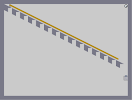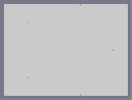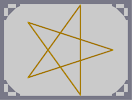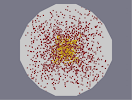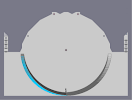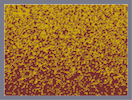Linar Death Star Lateral Equinox EXperimneto Blue Gene Obliquity (1 of 5)

Pages: (0)

its evil either way... i know
It's not "Satinism" you noobs! It's "Satanism"! Get it right! And the pentagram is only evil when upside down, like this: http://upload.wikimedia.org/wikipedia/commons/c/c4/Pentagram4.png

It is good when like this: http://adoptanamerican.com/version/imgs/pentagram.jpg

As for the map, good job. It's perfectly made! Well done, 5/5.

### um...

right...ok...

i dont think thats what this was ment to be...

666/5

666/5

### i dont know...

that star is the pentogram wich is satins symbol

satanism???
what satanism???

### wtf

wats with da satinism on this site

### um...

so???

im allowed to add afterthoughts, theres no edit function, so your just gonna have to live with it...

### wow nemetacyst

3 posts in a row!

dont mind me...

### basically

what im saying is i do understand...and because you did take the time to make it work perfectly, or almost prefectly, i wont hurt its rating cuz i dont really think its art...

### unless

well you could make it hard...

but with some decent math equations...it becomes simplistic...which i can tell you used, cuz the only part of the circle thats not done are the parts a graph has the most problems with getting points at...lol...

### ....

i know some programming codes, and no, its really not as hard, nowhere NEAR as hard...but i wont rate it...

### Of

course it was programmed, most of my recenpt levels have been partially programmed.. But you have to understand this: writing the code takes as much harship as making it (sometimes). So please consider that. Thanks for waiting nemetacyst.

### i laugh

at anybody who thinks this was done by hand...
and if it WAS done by hand, i must laugh at bobshike, becuase thats a colossal waste of time...

i dont believe it should be ont he top page of numa, but it is quite impressive, so i wont rate until i know of it was truely done by hand or not (i really doubt it)

### 5\5

THANKS!! i tried to load it...cool

### aslo

my next idea is sorta somming frome this one, but not compleatly. I also don't use every space available when making things. I like to just have a border and not everything perfect.

### I'm not jellous

actualy im not. I didn't say this was crap either. It is that these are "exploration of what can be made using Ned." I may have a diffrent Ned program than you do, but i still think that using any sort of or variation of Ned should be catagorized as automatically generated maps. That is all im's saying. Ill also make another map to prove that i can make a perfect curcle with only paint(I have 4 brothers and we take turns. It stinks). I am soury for wrighting with such a negitine tone, But u are using a Ned.

### Nice

But you would not need a program to do this. If you did this with time & patience, I would rate it a 4.5/5, but you get a 3.5/5 because all you had to do was connect the dots.

### PS

U SUK RAVENMAC UR JUST JEALOUS CUZ U SUK ASS

### holy crapola!

HOW ON EARTH DOES SUM1 HAVE THE KIND OF PATIENCE TO ALIGN MINES AND GOLD WITH A Z SNAP! I MEANS IT 100% PERFECT!! 5555/5555! LOL 5/5

### I don't agree

with your philosophy, but I do see what you're talking about. It's up to you to give me a 0; but I think that you should realize that even though the script generates the coordinates, I am the one who writes the script. And that isn't easy. So ultimately, it does take time and effort (and sweat and blood, how ever you take it). Anyways, if you think these maps are "crappy" go ahead. To me, these maps are an exploration of what can be made using Ned. And I'm sure the people who gave me a higher rating than 0 agree with me. (Don't take it harshly RavenMac).

### ...

same with the other side

### PS

your mine in the left most part of the curcle looks off.

### ...

I have given everything a 0/5 for everything you have done in Nart because of your "program" that "gives" you the cordinates. I think this is a automatically generating program, but it doesn't make the map its self. It just gives you cordinates you need and all you do is plug in what you want the things to be. That is why i have given you a 0/5 for everything in Nart. I also don't like show offs that come out of nowhere and places many things and get good rating on crapy things.

### This Lvl Is CRAZY!

that is so cool it makes pee taste good...
1000/1000!!!!!!!!!!!

### Ive never seen anything like this before!!!!!!!!

this is absolutely beautiful. 5 for you

### Effing Awesome!

geeeeess....crazy crazy crazy..... 5/5 always

Amazing!

### 5/5

i dunno how u do it.